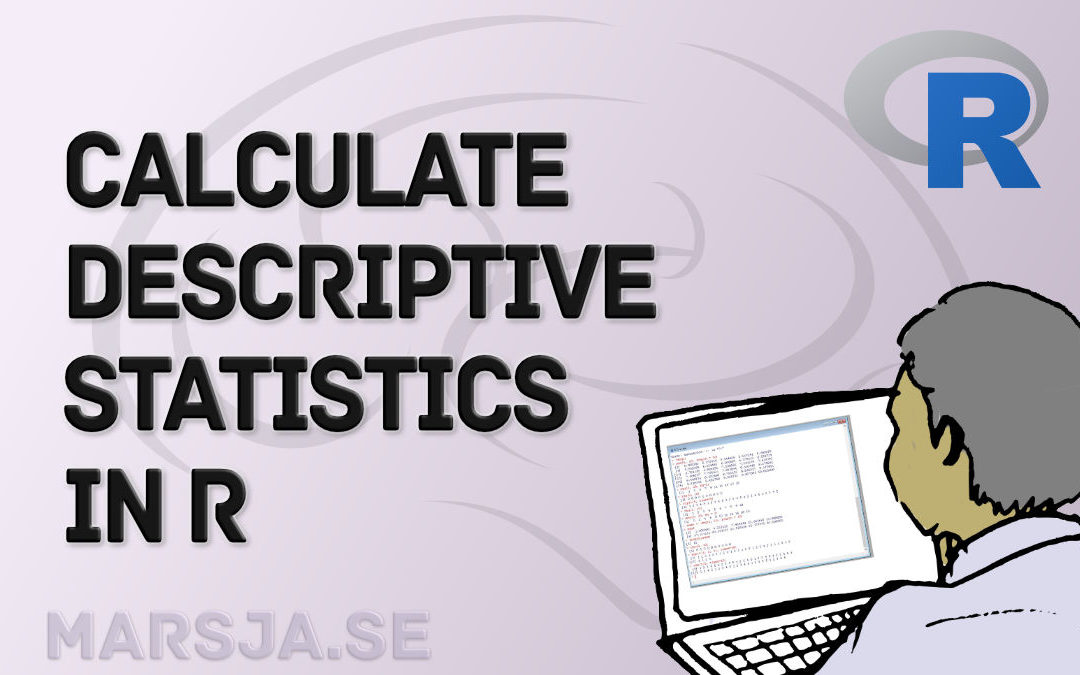In this PsychoPy tutorial, you will learn how to create the Psychomotor Vigilance Task (PVT) using some but not that much coding. However, as the setup for this test has a random interstimulus interval (ISI), we will create a Psychomotor Vigilance Test with a duration...## How to Calculate Descriptive Statistics in R the Easy Way with dplyr

In this tutorial, you will learn how to calculate descriptive statistics in R, a fundamental tool for data analysis. Descriptive statistics provide an overview of the key characteristics of a dataset, including measures of central tendency, variability, and...## How to Use Binder and R for Reproducible Research

In a previous post, we learned how to use Binder and Python for reproducible research. Now, we are going to learn how to create a Binder for our data analysis in R, so it can be fully reproduced by other researchers. More specifically, in this post, we will learn how...## How to Use Binder and Python for Reproducible Research

In this post, we will learn how to create a binder so that our data analysis, for instance, can be fully reproduced by other researchers. That is, here we will learn how to use a tool called Binder for reproducible research. In previous posts, we have learned how to...## OpenSesame Tutorial: How to use Image Stimuli

In this OpenSesame tutorial,l we will learn how to use images as stimuli and how to load the trials, including filenames, correct responses, and conditions, from a pre-generated CSV file. To follow this tutorial, you don’t need to know Python programming....## OpenSesame Tutorial – How to Create a Flanker Task

In this post, you are going to learn how to create a simple experiment using the free experiment building software OpenSesame. As I have previously written about, OpenSesame is an application based on Python for creating Psychology, Neuroscience, and Economics...